# 3 digit Addition without Regrouping

3 digit Addition without Regrouping – Example 1

Add the following:Explanation

Adding the digits at ones place,
4 + 3 = 7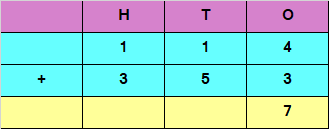Adding the digits at tens place,
1 + 5 = 6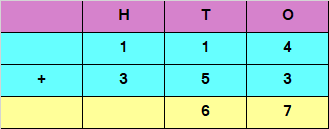Adding the digits at hundreds place,
1 + 3 = 4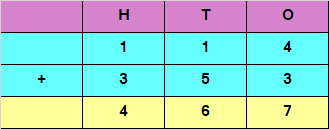Hence, the sum of the given numbers is 467

Example 2

Add the following: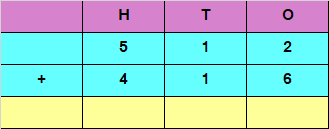Explanation

Adding the digits at ones place,
2 + 6 = 8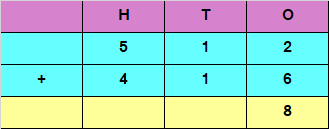Adding the digits at tens place,
1 + 1 = 2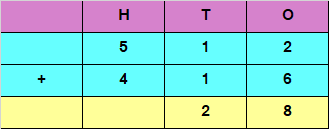Adding the digits at hundreds place,
5 + 4 = 9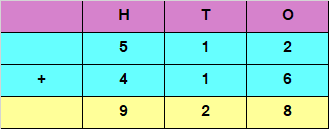Hence, the sum of the given numbers is 928